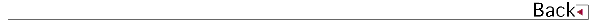\$B!!(B

# 2016\$BG/EY(B (2016.04\$B!A(B2017.03)

## \$B8&5fO@J8(B

• \$B8eF#(B \$BCi9-(B, \$B5WJ]2,(B \$B=S9((B, \$BBm8}(B \$BGn;N(B, \$B1+C+(B \$B=c(B, VLBI\$B4QB,NL\$rMQ\$\$\$?J|Aw1R@1\$N50F;7h(B, \$BEE;R>pJsDL?.3X2q!!O@J8;o(BB
• \$B@1Ln(B \$B=S2p(B, \$B>.NS(B \$B9-@,(B, \$B>.CS(B \$BE/;J(B, \$B668}(B \$B9@G7(B, \$B@nB<(B \$B@?<#(B, \$BB-N)(B \$B%"%[%m(B, \$B9u?\(B \$B@/?.(B, \$B;3K\(B \$B??G7(B, \$B3a86(B \$BM\$2p(B, \$BJL=j(B \$B9/B@O:(B, \$B4d^<(B \$B??3\$(B, \$B%&%#%s%I%W%m%U%!%\$%i\$H%>%s%G\$+\$i5a\$a\$?MpN.%(%M%k%.!<>C;6N(\$NHf3S(B, \$B5\$>]8&5f=j8&5fJs9p(B

## \$B<}O?O@J8(B

• \$BF#;^(B \$BH~Jf(B, \$B8eF#(B \$BCi9-(B, \$B1+C+(B \$B=c(B, Advanced two-way satellite frequency transfer by carrier-phase and carrier-frequency measurements, Journal of Physics: Conference Series
• \$B;3K\(B \$B??G7(B, \$B@nB<(B \$B@?<#(B, \$B2,K\(B \$BAO(B, \$BF#5H(B \$B9/;V(B, \$B9bJ,2rG=%&%#%s%I%W%m%U%!%\$%i\$K\$*\$1\$k%9%Z%/%H%k%Q%i%a!<%??dDj \$B!!!!!!!!!!!!!!(B
• \$B;3K\(B \$B??G7(B, \$B@nB<(B \$B@?<#(B, \$B@>B<(B \$B9L;J(B, \$B9bJ,2rG=(B1.3GHz\$BBS%&%#%s%I%W%m%U%!%\$%i\$N3+H/(B, 2016 International Symposium on Antennas and Propagation (ISAP2016)
• \$BCf>k(B \$BCRG7(B, \$B;3K\(B \$B??G7(B, \$B668}(B \$B9@G7(B, \$B5\$>]%l!<%@\$H%&%#%s%I%W%m%U%!%\$%i\$rMQ\$\$\$?J!0fJ?Ln\$K\$*\$1\$k9_1+J,I[\$N4QB,E*8&5f(B, 2016 International Symposium on Antennas and Propagation (ISAP2016) \$B!!!!!!!!!!!!!!(B
• \$B;3K\(B \$B??G7(B, \$B@nB<(B \$B@?<#(B, \$B@>B<(B \$B9L;J(B, \$B%l!<%@%\$%a!<%8%s%0\$rMQ\$\$\$?9bJ,2rG=%&%#%s%I%W%m%U%!%\$%i\$N3+H/(B, 2016 URSI Asia-Pacific Radio Science Conference

## \$B0lHL8}F,H/I=(B

• \$B8eF#(B \$BCi9-(B, \$B5WJ]2,(B \$B=S9((B, \$BBm8}(B \$BGn;N(B, \$B1+C+(B \$B=c(B, \$B1R@1J|Aw\$N?.9fCg2p\$K\$h\$k;~9oHf3SpJsDL?.3X2q(B2017\$BG/(B \$BAm9gBg2q(B
• \$B?yC+(B \$BLPIW(B, \$B1+C+(B \$B=c(B, \$B4d0f(B \$B9(FA(B, \$B5WEg(B \$BK(?M(B, \$B?9K\(B \$B>
• \$B8eF#(B \$BCi9-(B, \$B5WJ]2,(B \$B=S9((B, \$BBm8}(B \$BGn;N(B, \$B1+C+(B \$B=c(B, Domestic Time Transfer with Digital Broadcast Satellite Signals, Precise Time and Time interval Meeting
• \$B?yC+(B \$BLPIW(B, \$B1+C+(B \$B=c(B, \$B4d0f(B \$B9(FA(B, \$B5WEg(B \$BK(?M(B, \$B?9K\(B \$B>
• \$BBl@n(B \$BE/B@O:(B, \$B>.?K(B \$BE}(B, \$B?9K\(B \$B>l\$H%W%i%s%/%H%sJ,I[(B-\$B;31"2-1s5wN%3\$MN%l!<%@3\$0h\$K\$*\$1\$kJ*M}!&@8J*4QB,(B-, \$BF|K\3\$MN3X2q@>Fn;YIt(B \$B0lHL
• \$B@nB<(B \$B@?<#(B, \$BB@ED(B \$B905#(B, \$B2VEZ(B \$B90(B, \$B;3K\(B \$B??G7(B, \$B;V2l(B \$B?.BY(B, \$BLZ8M(B \$B9LB@(B, \$B0BED(B \$BE/(B, \$B8eF#(B \$BCi9-(B, \$B;T@n(B \$BN40l(B, \$B1+C+(B \$B=c(B, \$B:#B<(B \$BT"9/(B, \$BF#;^(B \$BH~Jf(B, \$B4d0f(B \$B9(FA(B, \$B?yC+(B \$BLPIW(B, \$B0f8}(B \$B=SIW(B, \$BCO>e%G%8%?%kJ|AwGH\$rMQ\$\$\$??e>x5\$?dDj\$H\$=\$N8m:9MW0x(B, \$BCO5eEE<'5\$!&CO5eOG@17w3X2q(B \$BAm2q5Z\$S9V1i2q(B \$B!J(BSGEPSS)
• \$B?yC+(B \$BLPIW(B, \$B1+C+(B \$B=c(B, \$B4d0f(B \$B9(FA(B, \$B5WEg(B \$BK(?M(B, \$B?9K\(B \$B>
• \$B@nB<(B \$B@?<#(B, \$BB@ED(B \$B905#(B, \$B2VEZ(B \$B90(B, \$B;3K\(B \$B??G7(B, \$B;V2l(B \$B?.BY(B, \$BLZ8M(B \$B9LB@(B, \$B0BED(B \$BE/(B, \$B8eF#(B \$BCi9-(B, \$B;T@n(B \$BN40l(B, \$B1+C+(B \$B=c(B, \$B:#B<(B \$BT"9/(B, \$BF#;^(B \$BH~Jf(B, \$B@PDE(B \$B7rB@O:(B, \$B4d0f(B \$B9(FA(B, \$B?yC+(B \$BLPIW(B, \$B0f8}(B \$B=SIW(B, Water vapor estimation using the propagation delay of digital terrestrial broadcasting waves, 2016 International Symposium on Antennas and Propagation
• \$BBm8}(B \$BGn;N(B, \$B8eF#(B \$BCi9-(B, \$BCf@n(B \$B;K4](B, \$B@.ED(B \$B=(\$B86;R;~7W1?HB\$K\$*\$1\$kAjBPO@8z2L\$NJd@5(B, \$BF|K\B,CO3X2q(B
• \$BBm8}(B \$BGn;N(B, \$B1+C+(B \$B=c(B, \$B8eF#(B \$BCi9-(B, \$BAjED(B \$B@/B'(B, \$B%Q%C%7%V%l!<%@\$K\$h\$kCOI=JQF04F;k(B, \$BF|K\B,CO3X2q(B
• \$B@nB<(B \$B@?<#(B, \$BB@ED(B \$B905#(B, \$B2VEZ(B \$B90(B, \$B;3K\(B \$B??G7(B, \$B;V2l(B \$B?.BY(B, \$BLZ8M(B \$B9LB@(B, \$B0BED(B \$BE/(B, \$B8eF#(B \$BCi9-(B, \$B;T@n(B \$BN40l(B, \$B1+C+(B \$B=c(B, \$B:#B<(B \$BT"9/(B, \$BF#;^(B \$BH~Jf(B, \$B@PDE(B \$B7rB@O:(B, \$B4d0f(B \$B9(FA(B, \$B?yC+(B \$BLPIW(B, \$BCO>e%G%8%?%kJ|AwGH\$rMQ\$\$\$??e>x5\$4QB,]3X2q(B \$B=U5(Bg2q(B
• \$BBm8}(B \$BGn;N(B, \$B8eF#(B \$BCi9-(B, \$BF#;^(B \$BH~Jf(B, \$BCf@n(B \$B;K4](B, \$B@.ED(B \$B=(>86(B \$B7rM4(B, \$B:#B<(B \$BT"9/(B, \$B0KEl(B \$B9(G7(B, \$B1+C+(B \$B=c(B, \$B2VEZ(B \$B\$f\$&;R(B, Relativistic Effect Correction for Clock Transport, EFTF
• \$B;3K\(B \$B??G7(B, \$B@nB<(B \$B@?<#(B, \$B@>B<(B \$B9L;J(B, \$B2,K\(B \$BAO(B, \$BF#5H(B \$B9/;V(B, \$B%&%#%s%I%W%m%U%!%\$%i\$K\$*\$1\$k?.9f=hM}(B-\$B%j%"%k%?%\$%`%G!<%?=hM}\$H%9%Z%/%H%k%Q%i%a!<%??dDj(B-, \$BF|K\5\$>]3X2q2-Fl;YIt8&5fH/I=2q(B
• \$B;3K\(B \$B??G7(B, \$B@nB<(B \$B@?<#(B, \$B@>B<(B \$B9L;J(B, \$B>pJsDL?.8&5f5!9=\$K\$*\$1\$k9bJ,2rG=(B1.3GHz\$BBS%&%#%s%I%W%m%U%!%\$%i\$N3+H/(B, \$BBh(B11\$B2s9R6u5\$>]8&5f2q(B
• \$B;3K\(B \$B??G7(B, \$B@nB<(B \$B@?<#(B, \$B@>B<(B \$B9L;J(B, \$B%&%#%s%I%W%m%U%!%\$%iMQ%G%8%?%k]3X2q(B2016\$BG/EY=U5(Bg2q(B
• \$BCf>k(B \$BCRG7(B, \$B;3K\(B \$B??G7(B, \$B668}(B \$B9@G7(B \$BJ!0fJ?Ln\$K\$*\$1\$k@>Iw;~\$N9_1+J,I[\$KBP\$9\$k2]3X2q(B2016\$BG/EY=U5(Bg2q(B

## 1990\$B!A(B1991\$B!d(B1992\$B!d(B1993\$B!d(B1994\$B!d(B1995\$B!d(B1996\$B!d(B1997\$B!d(B1998\$B!d(B 1999\$B!d(B2000\$B!d(B2001\$B!d(B2002\$B!d(B2003\$B!d(B2004\$B!d(B2005\$B!d(B2006\$B!d(B2007\$B!d(B 2008\$B!d(B2009\$B!d(B2010\$B!d(B2011\$B!d(B2012\$B!d(B2013\$B!d(B2014\$B!d(B2015\$B!d(B2016\$B!d(B2017